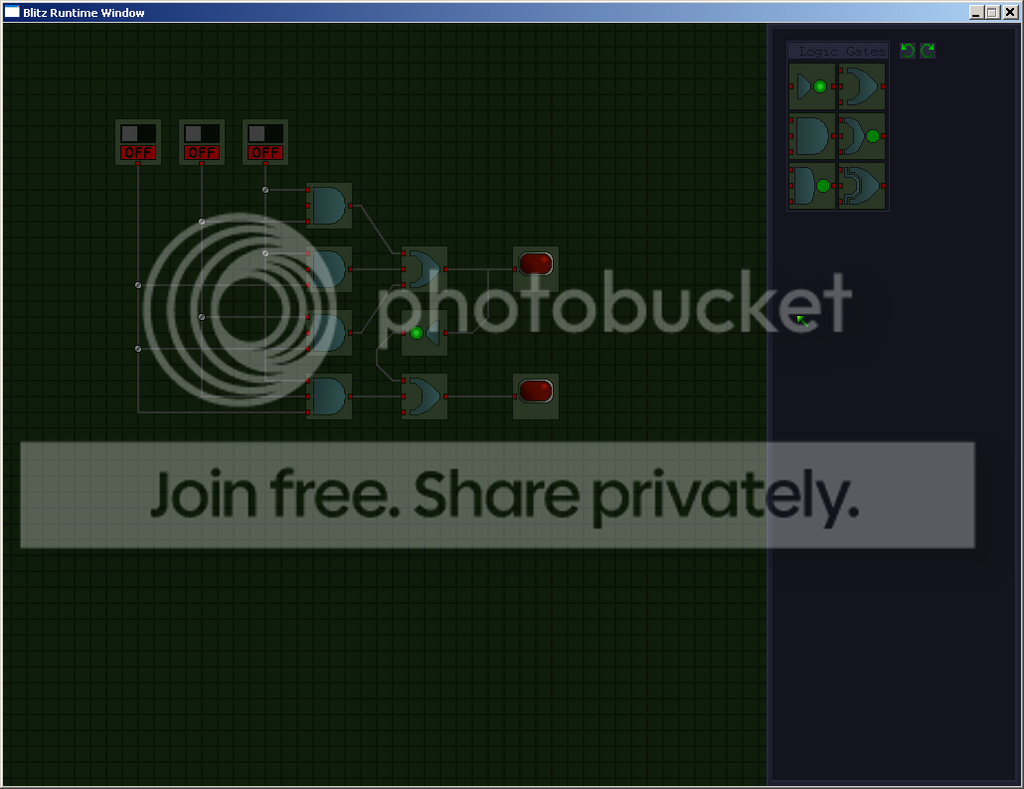-=+=- -=+=- -=+=- -=+=- -=+=- -=+=- -=+=- -=+=- -=+=- -=+=- -=+=- -=+=- -=+=- -=+=- -=+=- -=+=- -=+=- -=+=- -=+=- -=+=- -=+=- -=+=- -=+=- -=+=- -=+=- -=+=- -=+=- -=+=- -=+=- -=+=- (c) WidthPadding Industries 1987 0|432|0 -=+=- -=+=- -=+=- -=+=- -=+=- -=+=- -=+=- -=+=- -=+=- -=+=- -=+=- -=+=- -=+=- -=+=- -=+=- -=+=- -=+=- -=+=- -=+=- -=+=- -=+=- -=+=- -=+=- -=+=- -=+=- -=+=- -=+=- -=+=- -=+=- -=+=-

mike_gRPG Game

12th April 2007

Version 0.01

Heres a very early working version. You can do some stuff like equip items, eat food, use keys to unlock doors, and set off pressure plates.

Key controls for actions are:
L : look
O : open
E : eat
U : use
D : drop
Right clicking <snip>

mike_gLogic Box

8th November 2006this is just a screenie of a little puzzle game I started making last night.

Basically you will try to make curcuits that do different things, like the one I made above, using<snip>

More - Older Posts